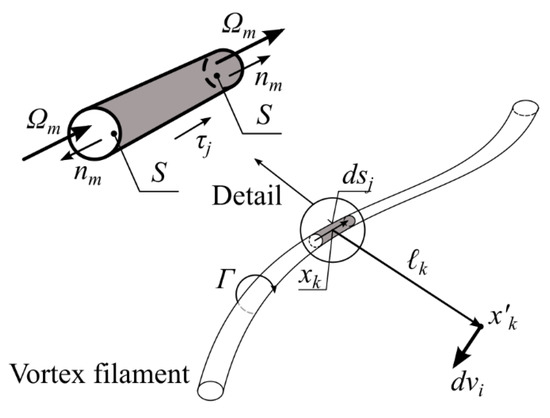Article

# Analytical Formula for the Mean Velocity Profile in a Pipe Derived on the Basis of a Spatial Polynomial Vorticity Distribution

Faculty of Mechanical Engineering, University of Technology Brno, Technická 2896/2, 616 69 Brno, Czech Republic
Academic Editors: Inmaculada Pulido-Calvo and Giuseppe Pezzinga
Water 2021, 13(10), 1372; https://doi.org/10.3390/w13101372
Received: 26 March 2021 / Revised: 23 April 2021 / Accepted: 10 May 2021 / Published: 14 May 2021
(This article belongs to the Section Hydraulics and Hydrodynamics)
The derivation of the mean velocity profile for a given vorticity distribution over the pipe cross-section is presented in this paper1. The velocity profile and the vorticity distribution are axisymmetric, which means that the radius is the only variable. The importance of the vortex field for the flow analysis is discussed in the paper. The polynomial function with four free parameters is chosen for the vorticity distribution. Free parameters of this function are determined using boundary conditions. There are also two free exponents in the polynomial. These exponents are determined based on the comparison of this analytical formula with experimental data. Experimental data are taken from the Princeton superpipe data which consist of 26 velocity profiles for a wide range of Reynolds numbers (Re). This analytical formula for the mean velocity profile is more precise than the previous one and it is possible to use it for the wide range of Reynolds number <31,577; 35,259,000>. This formula is easy to use, to integrate, or to derivate. The empirical formulas for the profile parameters as a function of Re are also included in this paper. All information for the mean velocity profile prediction in the mentioned Re range are in the paper. It means that it is necessary to know the average velocity v(av), the pipe radius R, and Re to be able to predict the turbulent mean velocity profile in a pipe. View Full-Text
Show FiguresFigure 1

MDPI and ACS Style

Štigler, J. Analytical Formula for the Mean Velocity Profile in a Pipe Derived on the Basis of a Spatial Polynomial Vorticity Distribution. Water 2021, 13, 1372. https://doi.org/10.3390/w13101372

AMA Style

Štigler J. Analytical Formula for the Mean Velocity Profile in a Pipe Derived on the Basis of a Spatial Polynomial Vorticity Distribution. Water. 2021; 13(10):1372. https://doi.org/10.3390/w13101372

Chicago/Turabian Style

Štigler, Jaroslav. 2021. "Analytical Formula for the Mean Velocity Profile in a Pipe Derived on the Basis of a Spatial Polynomial Vorticity Distribution" Water 13, no. 10: 1372. https://doi.org/10.3390/w13101372

Find Other Styles
Note that from the first issue of 2016, MDPI journals use article numbers instead of page numbers. See further details here.

1